# Community

Blog Events Webinars Tutorials Forum
×
Community Blog Learning Machine Learning, Part 2: Algorithms and Techniques

# Learning Machine Learning, Part 2: Algorithms and Techniques

In most Machine Learning courses, regression algorithms are the first to be introduced for two reasons: Regression algorithms are relatively simple,

The previous blog post, Introduction to Machine Learning, presented the Machine Learning concept. Now, let’s discuss representative methods used in the technology.## Regression Algorithms

In most Machine Learning courses, regression algorithms are the first to be introduced for two reasons:

1. Regression algorithms are relatively simple, and discussing them allows you to slide easily from statistics on to Machine Learning.
2. Regression algorithms provide the foundation for some of the powerful algorithms to be discussed late

There are two regression algorithm subtypes: linear regression and logistic regression.

We have already touched on linear regression via the House Price Problem. Let’s figure out how to find the straight line that best fits all the data.

The "least squares" method is used to solve this problem. The thinking behind this method is: to find the line that best represents a set of data; and find

the line that most closely follows the observed data points in the data set. Not all of the data points lie exactly along a straight line, so the best fit line is

the one with the least deviation from those points. To minimize the deviation, find a straight line that minimizes the sum of the squares of all the

distances between the points and the line. The least squares method converts an optimization problem into an extreme function value problem. In

mathematics, we can find the extreme values of a function by looking at the point where the derivative is 0. However, this method is not suitable for

computers because they may fail to solve the problem or the computation may be too intensive.

In computer science, there is a specialized discipline called numerical computation. It strives to increase accuracy and efficiency when computers run

various computations. For example, the famous gradient descent method and Newton method are classic numerical computation algorithms. They are

also well-suited to find extreme values of functions. The gradient descent method is one of the simplest and most effective methods for solving regression

1. The neural networks and recommendation algorithms to be discussed later have linear regression factors, and the gradient descent method is

applied in other algorithms.

Logistic regression algorithms are similar to linear regression algorithms. However, the types of problems addressed by linear regression are different

from those addressed by logistic regression. Linear regression deals with numerical problems with final predictions being numbers, such as a house price.

Logistic regression, on the other hand, is used in classification algorithms. The predictions made out of logistic regression are discrete classes like logistic

regression is often used to determine a spam email or if a user will click on an advertisement.

In practice, logistic regression simply adds a Sigmoid function to the results computed by linear regression. This function converts the numerical result

into a probability between 0 and 1 (images of the Sigmoid function are not very intuitive, but you need to understand that larger values are closer to 1

and smaller values are closer to 0). Next, we make a prediction based on this probability. For example, if the probability is greater than 0.5, we can classify

an email as spam or judge a tumor to be malignant. For better understanding, refer to the logistic regression algorithm shown as a classification line in

the following figure.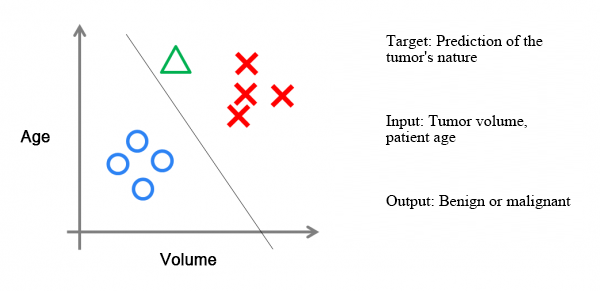Assume there is a set of data on cancer patients. Some of these patients' tumors are benign (blue circles), while some are malignant (red Xs). The

tumors' red or blue markings are data "tags." Each data point includes two features: patient's age and tumor size. You can map these two features and

the data tags in two-dimensional space.

Now, there is a new data point (green triangle) to help determine if this tumor is malignant or benign. To do this, you can train a logistic regression

model based on the red and blue data points. This is the classification line shown in black. The green point is to the right of the classification line, so you

can determine that the tag should be red, indicating a malignant tumor.

The classification lines drawn by logistic regression algorithms are always linear (sometimes logistic regression uses non-linear lines, but these models

suffer from low efficiency when processing large volumes of data). Therefore, when a line dividing two classifications is not linear, logistic regression is

unable to express the situation adequately. Next, let's discuss the two most powerful and important algorithms used in ML. Both are used to find non-

linear classification lines.

## Neural Networks

Neural network algorithms (also called artificial neural networks or ANN) are used widely in deep learning, neural networks have returned. They are

one of the most powerful Machine Learning algorithms.

Neural networks originated from the study of brain mechanisms. Biologists used neural networks to simulate the brain. Then, Machine Learning

academics used neural networks to perform Machine Learning experiments and found that they performed quite well for visual and audio recognition.
The specific learning mechanisms of neural networks decompose and integrate data. In the famous Hubel-Wiesel experiment, researchers investigating

the visual analysis mechanisms of cats found this to be the mechanism at work.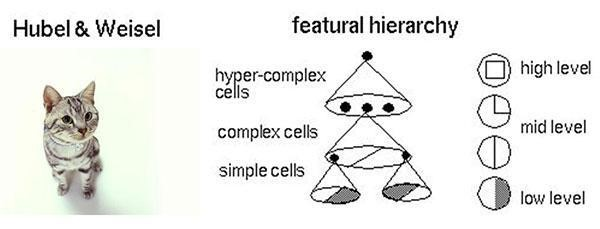For instance, a square features four polylines and then enters the next layer of visual processing. Each of the four neurons is used to process one

1. Each polyline further decomposes into two straight lines, and then the straight lines decompose into two black-and-white planes. Therefore, a

complex image converts into data when entering neurons. After being processed by the neurons, it is integrated. Finally, the brain sees the resulting

1. This is the brain's visual recognition mechanism as well as the working mechanism of neural networks.

Let's now look at the logical structure of a simple neural network. This network can be divided into input, hidden, and output layers. The input layer

receives signals; the hidden layer analyzes and processes data; and the final result is integrated into the output layer. In each layer, the circles represent

processing units. These can be thought of as simulated neurons. Several processing units combine to form a layer, and several layers form a network. The

result is a neural network.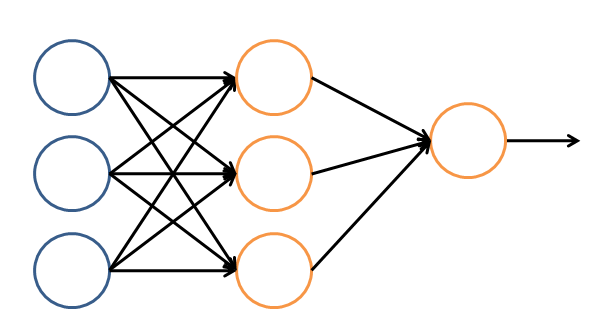In a neural network, each processing unit is a logistic regression model that receives input from the upper layer and outputs prediction results to the

next layer. Through this process, neural networks can accomplish complex, non-linear classification.
The following figure demonstrates a famous application of neural networks in the image recognition field. The program is called, LeNet, and the neural

network is constructed with multiple hidden layers. LeNet can recognize numbers written in different ways to achieve a high degree of accuracy and

robustness.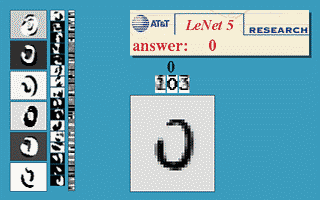The square at the center shows the images put into the computer. At the top, the red number after "answer" is the computer's output. The three

columns on the left-hand side of the image show the output of the neural network's three hidden layers. As the layers become deeper, there is less detail,

like we see that , the basic processing in layer three has reached only linear detail.

In the 1990s, the development of neural networks encountered a bottleneck because even with BP algorithm acceleration. therefore, in the late

1990s, SVM algorithms replaced neural networks.

## Support vector machine (SVM)

Support vector machine algorithms originated from the statistical learning field and came into their own as a classic algorithm in the ML field.
In a sense, SVM algorithms are enhancements of logistic regression algorithms. By providing logistic regression algorithms with stricter optimization

conditions, SVM algorithms draw better classification boundary lines than traditional logistic regression.

When combined with a Gaussian "core," SVM can express extremely complex classification borders to produce better classification results. The "core"

is a special function. The most typical feature of such a function is that it can map data from low-dimensional space to high-dimensional space.

&nbps;&nbps; Here is an example: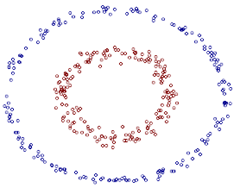Now, the issue becomes how to draw a circular classification border in two-dimensional (2-D) space, which is difficult. However, use the "core” to

map the 2-D data to three-dimensional (3-D) space. Then, use a linear plane to achieve similar results. Hence, a non-linear classification border in 2-D

space is equivalent to a linear border in 3-D space. You can therefore use a simple line to divide data in 3-D space and achieve the effect of a non-linear

division in 2-D space. The following is a section of 3-D space.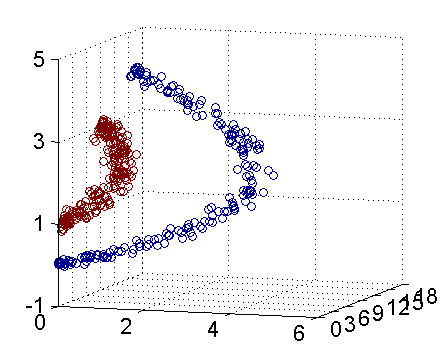SVM algorithms are mathematical ML algorithms (comparatively, neural networks have a biological component). The core step of the algorithm offers

further proof that data mapped from low-dimensional space to high-dimensional space does not increase the complexity of the final computation.

Therefore, SVM algorithms help maintain computational efficiency and deliver improved classification results.

## Clustering Algorithms

For all the previously mentioned algorithms, one prominent characteristic is that the training data contains tags and trained models can predict tags

for unknown data. In the following algorithms, the training data does not include tags, and the algorithms strive to infer these data tags through training.

These algorithms have a common name: unsupervised algorithms (algorithms that use tagged data are supervised algorithms). Typical unsupervised

algorithms are clustering algorithms. Returning to 2-D data, you can say that each data point has two features.

How can you use a clustering algorithm to mark them with various tags? Simply put, a clustering algorithm computes the distance between

groupings and based on these distances, divides data points into multiple groups. The most typical example of a clustering algorithm is the K-Means

algorithm.

## Descending Dimension Algorithms

A descending dimension algorithm is a type of unsupervised algorithm. It reduces data from a high-dimensional level to a low-dimensional level.

Dimensions represent the size of features that characterize the data. For instance, house price data may have four features: house length, width, area, and

number of rooms. Therefore, it is four-dimensional (4-D) data. The features of length and width overlap with the area feature because area = length x

1. Using a descending dimension algorithm, you can remove redundant information and reduce the features to area and number of rooms to

compress 4-D data into 2-D data.

You can use mathematics to prove that an algorithm compresses the number of dimensions while retaining the maximum amount of information.

Thus, descending dimension algorithms are still advantageous.

They are primarily used to compress data and enhance the efficiency of other ML algorithms. Another advantage is visualization. For example, if you

compress five-dimensional (5-D) data into 2-D data, the data can be visualized on a 2-D plane. Principal Component Analysis (PCA) algorithms are the

most typical example of a descending dimension algorithm.

## Recommendation Algorithms

Recommendation algorithms are very popular in the ML industry and widely used by e-commerce companies, such as Amazon, Tmall, and Jingdong.

The primary characteristic of recommendation algorithms is that it automatically recommends things that most interest a user to increase purchase rates.

There are two main types of recommendation algorithms.

Algorithms that give recommendations based on item content.

These algorithms recommend items to a user that are similar to their recent purchase. They require each item to have several tags in order to find

items similar to the user's purchased items. The advantage of these recommendations is their high degree of relevance.

Recommendation algorithm that gives recommendations based on similar users.

They show the user recommendations based on items purchased by other users with the same interests. For example, if user A has purchased items B

and C and the algorithm discovers that user D has the same interests as user A, and has bought item E, it will recommend item E to user A.

Both types of recommendation algorithms have their respective advantages and disadvantages. In e-commerce applications, sellers use a mix of both

1. Among recommendation algorithms, the most famous are the collaborative filtering algorithms.

## Others

In addition to the algorithms previously mentioned, many others are used in Machine Learning, such as Gaussian discriminant, naive Bayes, and

decision tree algorithms. Howevjer, the six algorithms discussed previously are the most widely used, have the greatest impact, and are the most

representative types.

Here is a summary of the algorithms:

• Supervised algorithms (with tags): linear regression, logistic regression, neural networks, and SVM
• Unsupervised algorithms (without tags): clustering algorithms and descending dimension algorithms
• Special algorithms: recommendation algorithms

In addition to these algorithms, there are some algorithms whose names are often mentioned in the Machine Learning field. However, these are not

true Machine Learning algorithms. Instead, they originated from attempts to solve some sub-problems. Among these "sub-algorithms," the most

representative is the gradient descent method, mainly used in linear regression, logistic regression, neural networks, and recommendation algorithms;

Newton method, used in linear regression; BP algorithms, used in neural networks; and SMO algorithms, used in SVM.

## Conclusion

In this blog post, we learned about algorithms that help implement ML functions. These include regression algorithms, neural networks, SVM,

clustering algorithms, descending dimension algorithms, and recommendation algorithms. In the next blog post, Machine Learning Applications, we look

at its applications in related fields, including big data, artificial intelligence, and deep learning.

0 0 0
Share on

# Related Products

• ##AI Acceleration Solution

Accelerate AI-driven business and AI model training and inference with Alibaba Cloud GPU technology

• ##EasyDispatch for Field Service Management

Apply the latest Reinforcement Learning AI technology to your Field Service Management (FSM) to obtain real-time AI-informed decision support.

• ##AIRec

A high-quality personalized recommendation service for your applications.

• ##Sports Solution

From immersive stadiums to data analytics, our sports solutions empower teams, athletes, and fans through the digital transformation of the sports industry